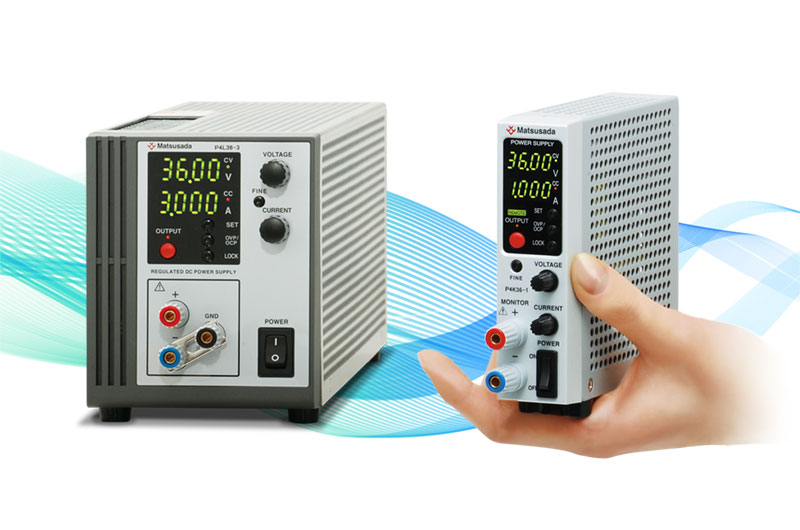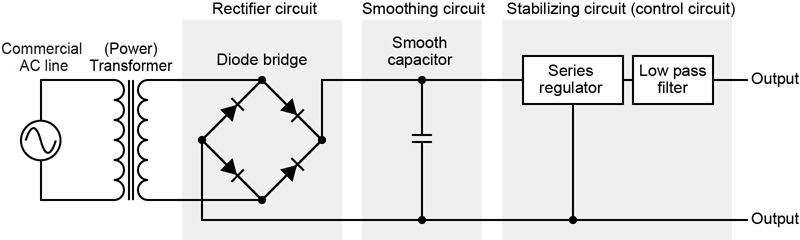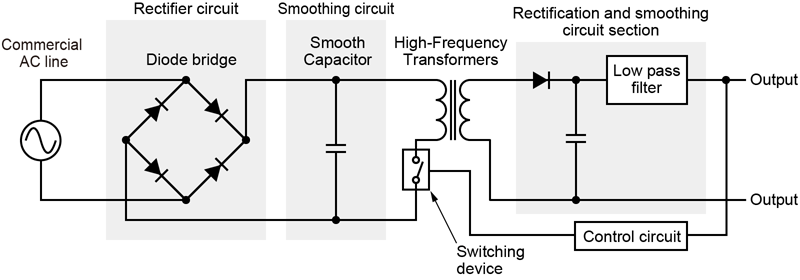Searching...

# Tech Tips## Mechanism of linear power supply and switching power supply

Both linear and switching power supplies provide a stable direct current (also abbreviated as "direct current" or "DC").
DC is used in the circuits of most electronic devices, such as smartphones, computers, air conditioners, and factory robots. Because each type of device and internal circuit of such electronic equipment require different voltages, a direct current source appropriate for each voltage is necessary.
The linear power supply was invented first. Linear power supplies are also known as series power supplies. In a linear power supply, a transformer consisting of an iron core and coil is used to reduce the voltage to an incoming alternating current (AC). The voltage is then rectified by a diode in the rectifier circuit and smoothed by a capacitor in the smoothing circuit to provide a stable voltage.
The voltage output from the rectifier circuit is a series of positive peaks of a sine wave, which is not a suitable stable current. Therefore, the voltage is leveled using a smoothing circuit consisting of a capacitor, and a stabilizing circuit (control circuit). There are two types of control circuits: shunts and series circuits. Both methods monitor and control the output DC voltage to maintain a constant value. The difference between the input and output voltages generates heat; therefore, a large heat sink is required.
A linear power supply requires a dedicated AC transformer based on input voltage, output voltage, and power. Therefore, each device had a fixed capacity and required a power supply with a dedicated transformer for each application.In contrast, switching power supplies feature rectification and smoothing prior to voltage regulation. A chopper is used to convert the incoming voltage to a high-frequency pulse train. By turning the switch on and off at a high speed, the rectified current is treated as a pseudo alternating current (AC) with a pulse wave, and the voltage is regulated using a high-frequency transformer.## Comparison of linear power supply and switching power supply

The typical difference between a linear power supply and a switching power supply is the amount of noise and the size of the power supply. As mentioned above, the switching power supply repeatedly turns the switch on and off at high speed. This causes noise owing to switching. Linear power supplies produce less noise than switching power supplies.
As mentioned above, the switching power supply repeatedly turns the switch on and off at high speed. This causes noise due to switching. In terms of noise, linear power supplies produce less noise than switching power supplies.
If we compare a linear power supply and a switching power supply that produces the same output, the noise of the linear power supply (R4G18-2) is 0.5 mVrms and 1 mArms as provided in the datasheet specifications. However, the switching power supply (R4K18-2) is about 5 mVrms and 5 mArms.
In other words, the voltage noise of the switching power supply is ten times greater and the current noise is five times greater than that of a linear power supply.
The next step was to determine the size of the power supply. The linear power supply was small; however, the switching power supply was smaller. This is due to the size of the transformer. If the voltage varies by the same amount, the transformer will be smaller at higher frequencies.
In a linear power supply, the frequency input from an outlet or another source is input directly to the transformer, whereas in a switching power supply, the current after rectification is sent to the transformer as a high-frequency pulse. Therefore, the transformer is small.
For example, a power supply that can output a DC voltage of 18 V, the linear power supply (R4G18-2) measures 124 × 84 × 325 mm (H × W × D) and weighs approximately 3 kg. In contrast, the switching power supply (R4K18-2) measures 124 × 35 × 128 mm (H × W × D) and weighs approximately 500 g.
They are both the same height, but the linear power supply is 2.4 times wider, 2.5 times deeper, and weighs six times more than the switching power supply. This is because transformers use an iron core, and the size of the transformer affects its weight.
Switching power supplies came into general use around 1990, much after linear power supplies. The old AC adapters are very large and heavy because they use linear power supplies.
In recent years, gallium nitride (GaN) power supplies with low energy losses have been commercialized.
GaN power supplies are semiconductors made of gallium nitride, which is similar to conventional silicon-based semiconductors. Transistors using GaN have lower power loss than conventional transistors.
The development of these new technologies has led to the creation of smaller and more powerful switching power supplies.

## Other differences

There are other differences between linear and switching power supplies in addition to noise and magnitude, which are explained below.

Switching power supplies have lower energy-conversion losses.

NASA originally developed switching power supplies for space applications. Therefore, they were designed to provide high energy efficiency. Switching power supplies is more efficient because linear power supplies lose more energy as heat.

Linear power supply responds more quickly to load fluctuations

The output of the switching power supply was controlled by the control circuit. In contrast, linear power supplies are controlled by the response of the regulator circuit. Therefore, the linear power supply exhibits a faster response to load fluctuations.

If the output power is small, the linear power supply can be produced at a lower cost, and if it is large, the switching power supply becomes the less expensive option.

Linear power supplies are less expensive because of their simpler structures. However, as mentioned above, linear power supplies have lower efficiency and generate more heat than switching power supplies; therefore, when power consumption increases, measures must be taken for the power consumed by the linear power supply itself and the heat emitted by it.
Therefore, as power consumption increases, the total cost of the switching power supply becomes less than that of a linear power supply. As an approximate guide, linear power supplies can be operated at a lower cost if the power is lower than 400 W.

The differences between linear and switching power supplies are summarized below.

Linear power supply Switching power supply
Noise (electric) Small Large
Circuit (electric) Simple Complex
Power supply unit size Big and heavy Small and light
Conversion loss (heat generation) Large Small
Response Fast Slightly slower
Low cost Advantageous in low-power power supply High-power (high-power) power supply

### Recommended products

Matsusada Precision offers both linear regulator-type DC power supplies and switching-type DC power supplies based on the application.